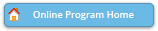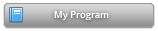#### Abstract Details

 Activity Number: 256 - Contributed Poster Presentations: Section on Statistical Learning and Data Science Type: Contributed Date/Time: Monday, July 29, 2019 : 2:00 PM to 3:50 PM Sponsor: Section on Statistical Learning and Data Science Abstract #307324 Title: Recursive Optimization Using Diagonalized Hessian Estimate and Its Application in EM Author(s): Shiqing Sun* and James C. Spall Companies: and Applied Physics Laboratory Keywords: Stochastic Optimization; EM algorithm; SPSA Abstract: Simultaneous perturbation stochastic approximation (SPSA) and its adaptive version (ASPSA) are two algorithms in stochastic recursive optimization problems, analogous to gradient descent and the Newton method. In this presentation, we propose an adaptive method that uses only diagonal elements of the Hessian estimates, which are generated from the same technique as in ASPSA, to rescale the estimates of gradients. Moreover, we apply our method in EM algorithm by approximating the Fisher information matrix matrix by the Hessian.\\ Our algorithm has the advantage that with dimension denoted as \$p\$, the modified algorithm involves \$O(p)\$ computational costs, which improves the computation complexity of second order optimization algorithms (usually \$O(p^3)\$). This modification improves models that need high-dimensional estimation, such as Bayesian neural networks and hidden markov models.\\ Furthermore, our method is suitable when the Fisher information matrix is difficult to compute in a high-dimensional inference case when the target distribution is complicated.\\ We state our results in the convergence part of the algorithm and presents its performance in numerical tests.

Authors who are presenting talks have a * after their name.